#The Wolfram Language:Fast Introduction for Programmers

All sections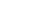Get Started

# AssignmentsVideo Version

Notes for Java programmers:

Since the Wolfram Language is dynamically typed, you don't need to specify a type when assigning variables.

Values can be assigned using =

In:=1`x = 7`
Out=1This is "immediate" assignment.

An alternative is delayed assignment, where the value is recomputed every
time it is needed:

In:=2`t := Now`

In:=3`t`
Out=3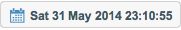In:=4`t`
Out=4Notes for Java programmers:

Java does not have delayed assignments. Achieving similar functionality would require a separate method call for each update.

Notes for Python programmers:

In the Wolfram Language, delayed assignments create variables that dynamically recompute their value every time you use them. Achieving similar functionality in Python would require the use of more complex promise objects.

Clear the assignments:

In:=1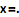`x =.`

In:=1`t =.`

Notes for Java programmers:

Using =. in the Wolfram Language is similar to setting a value to null in Java, but without the risk of NullPointerException.

Notes for Python programmers:

=. in the Wolfram Language works like Python's del operator.

Use Module to localize variables:

In:=2`Module[{a = 1}, a + 8]`
Out=2Notes for Java programmers:

Module introduces scoped variables similar to how methods in Java create locally scoped variables.

Notes for Python programmers:

Functions in Python create locally scoped variables similar to Module in the Wolfram Language.

It's conventional to start variable names with lowercase letters, reserving capitals for built-in objects: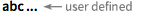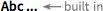Notes for Java programmers:

In Java, capitalized names specifically represent classes; in the Wolfram Language, capitalized names represent built-in constructs of any kind.

Notes for Python programmers:

In Python naming conventions, capitalized names specifically represent classes; in the Wolfram Language, capitalized names can represent built-in constructs of any kind.

Which of the following will NOT evaluate to 6?

Which of these could be a user-defined variable?

Which of the following clears any assignment for y?